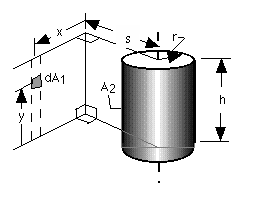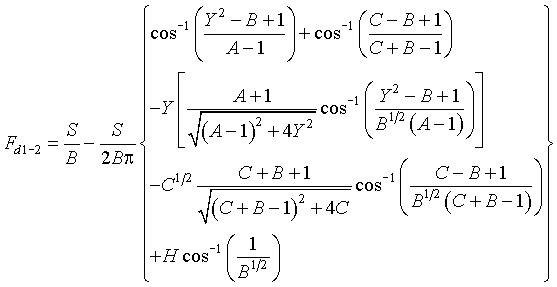>

# SECTION B Factors From Differential Elements to Finite Areas

## B-32: Element on plane to exterior of right circular cylinder of finite length. Plane does not intersect cylinder.Definitions: S=s/r; X=x/r; Y=y/r; H=h/r; A=X2+Y2+S2; B=S2+X2; C=(H-Y)2

Governing equation:To download a copy of this equation in MS Word 97 Format, click HERE.